DAY 21
0
Microsoft Azure

# 準備商品的收盤價

``````c = df["ClosePrice"]
``````

# 製作均線

``````# 製作均線
c_5 = c.rolling(5, min_periods=1).mean()
c_20 = c.rolling(20, min_periods=1).mean()
c_60 = c.rolling(60, min_periods=1).mean()

# 印出均線圖
c.plot()
c_20.plot()
c_60.plot()
``````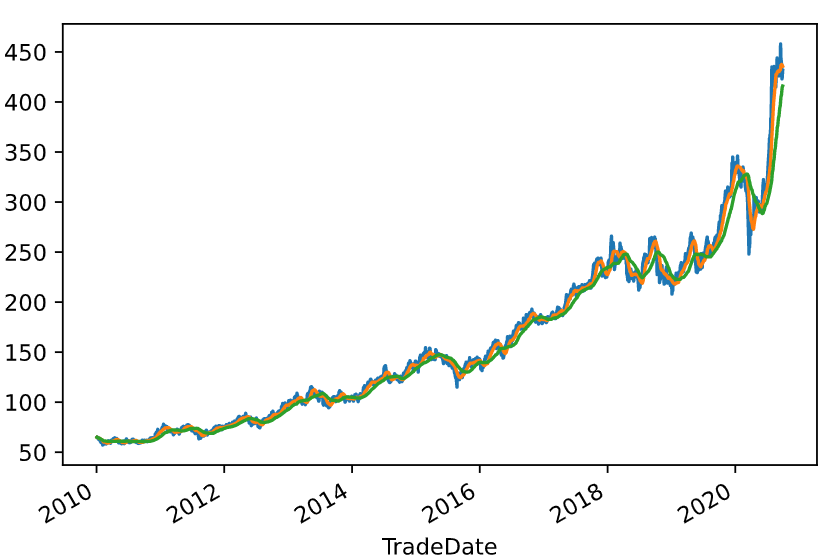# 策略比較

## 策略一

``````# 買入訊號
signal = (c > c_20)

# 回測並跟大盤比較
(c.shift(-1) / c)[signal].cumprod().plot(color='red')
(c.shift(-1) / c).cumprod().plot(color='blue')
``````## 策略二

20日均線比60日均線還要高時，買進台積電。

``````# 買入訊號
signal = (c_20 > c_60)

# 回測並跟大盤比較
(c.shift(-1) / c)[signal].cumprod().plot(color='red')
(c.shift(-1) / c).cumprod().plot(color='blue')
``````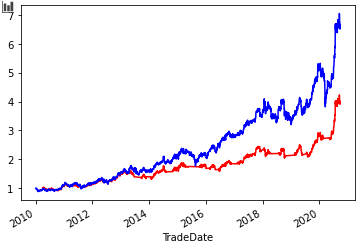## 策略三

60日均線比20日均線還要高時，買進台積電。

``````# 買入訊號
signal = (c_60 > c_20)

# 回測並跟大盤比較
(c.shift(-1) / c)[signal].cumprod().plot(color='red')
(c.shift(-1) / c).cumprod().plot(color='blue')
``````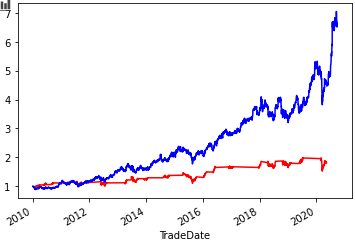## 策略四

``````# 買入訊號
signal = (c > c_60)

# 回測並跟大盤比較
(c.shift(-1) / c)[signal].cumprod().plot(color='red')
(c.shift(-1) / c).cumprod().plot(color='blue')
``````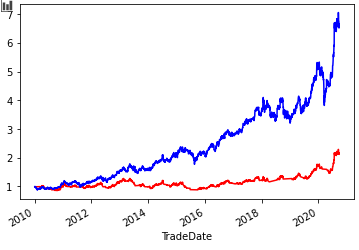## 策略五

``````# 買入訊號
signal = (c > c_5)

# 回測並跟大盤比較
(c.shift(-1) / c)[signal].cumprod().plot(color='red')
(c.shift(-1) / c).cumprod().plot(color='blue')
``````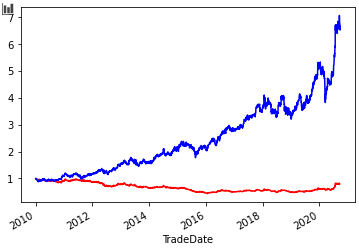## 策略六

5日均線比收盤價還要高時，買進台積電。

``````# 買入訊號
signal = (c < c_5)

# 回測並跟大盤比較
(c.shift(-1) / c)[signal].cumprod().plot(color='red')
(c.shift(-1) / c).cumprod().plot(color='blue')
``````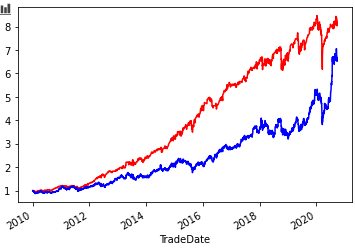# 觀看增長率狀況

``````pandas.DataFrame({'c':c, 'c_5':c_5, '增長率':c.shift(-1)/c, 'signal':signal})
``````